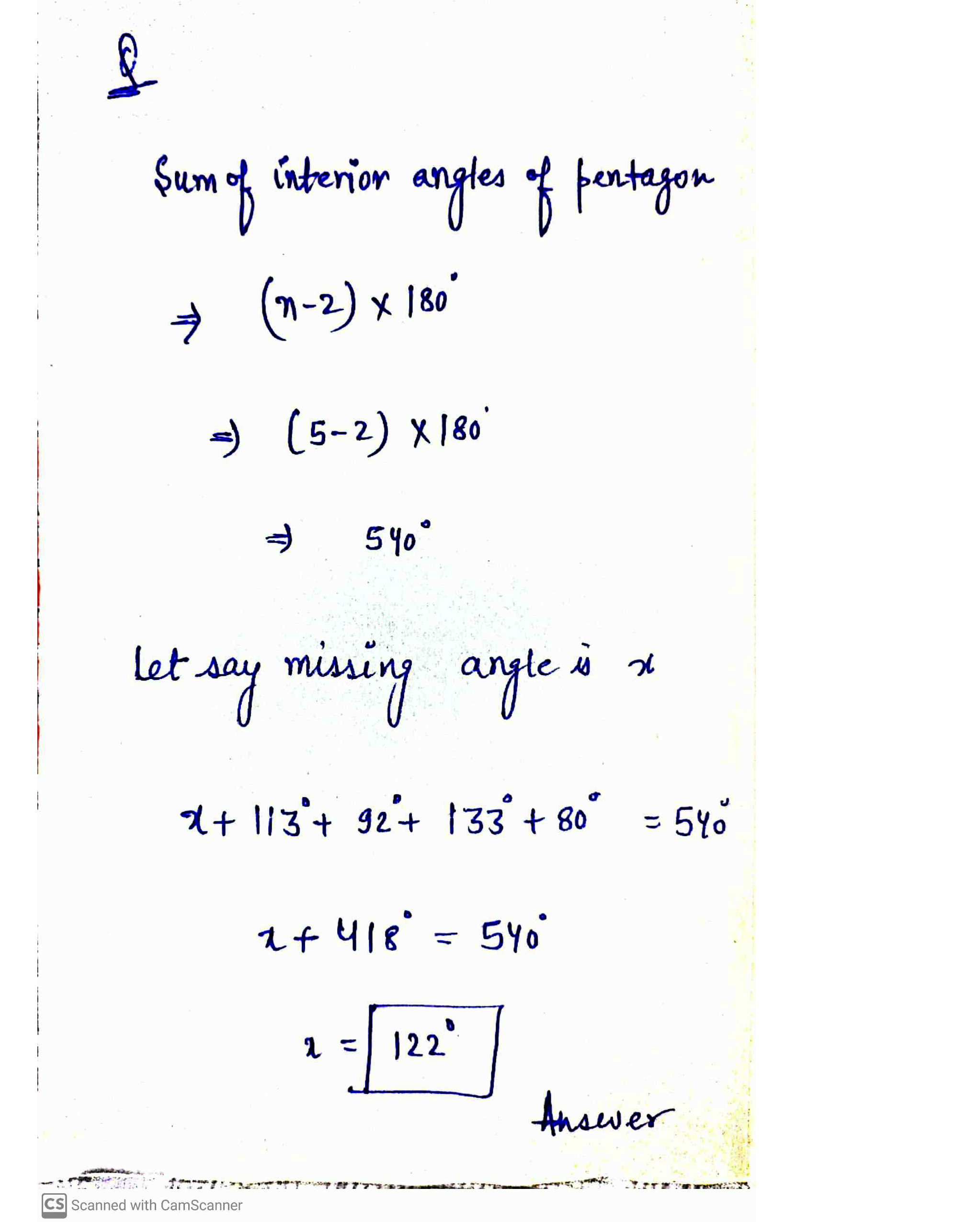Question

Geometry

-- Find the missing angle measures in each of the following polygons.Verified### Question 44851Geometry

8. Draw and describe tessellations.
Part I: A regular tessellation uses only one regular polygon to completely cover a surface with no overlaps or spaces. Draw a regular tessellation in the box below using only equilateral triangles, squares, or regular hexagons. (3 points)
Part II: A semi-regular tessellation uses more than one regular polygon to completely cover a surface with no overlaps or spaces. Name the polygons used in each semi-regular tessellation. (4 points; 2 points each)

### Question 44850Geometry

7. A line of symmetry divides a design so that every point on one side of the line coincides withal point on the other side of it. Determine how many lines of symmetry each figure below has.Then draw all the lines of symmetry. Show your work.

### Question 44849Geometry

Part I: The word BOOK has a horizontal line of symmetry when it is written in all capitalletters. Write two other words with four or more letters that have a horizontal line ofsymmetry. (2 points)
Part II: The letter Y has a vertical line of symmetry when it is written as a capital letter. Findfive other letters that have a vertical line of symmetry. Then draw each letter showing thelines of symmetry. Show your work. (2.5 points)
Part III: Rotational symmetry is the quality a design has if it maintains all characteristics when it is rotated about a point. Find five letters that have rotational symmetry. Draw each letter showing the point of symmetry. (2.5 points)

### Question 44848Geometry

5. You are assembling pieces of an iron gate to complete a fence. The finished gate will look likethe one below. In order to assemble the gate it is important to understand how the pieces arerelated .
: How are pieces 1 and 2 in the archway related? (1 point)
Part II: How are the rectangular sections 5 and 6 in the center of the gate related to each other? (1 point)

### Question 44847Geometry

4. In the list below, determine whether or not each example is a rigid motion. If it is a rigidmotion, identify the type of transformation.(6 points)

### Question 44846Geometry

3. Jeanne makes jewelry and has an idea for a new pair of earrings. She wants to make a sketchof her design, but only has a marker, a pencil, two pieces of paper, and a coffee mug. Use theseitems to construct the shape of the earring Jeanne is envisioning.
Step 1: Jeanne traced her coffee mug on her piece of paper. How might she use paper-folding to find the center of the circle? (2 points)
Step 2: Show how a second circle should be drawn in order to continue the construction.What can you say about the radii? Explain your work. (4 points)
Step 3: Describe the final steps in constructing the shape of the earring. Include a drawing.(2 points)

### Question 44845Geometry

2. The rules for constructions are based on the five basic postulates of Euclidean geometry.Complete the diagrams to show each postulate. (5 points; 1 point each)
A. First postulate: A straight line segment can be drawn between any two points.
B. Second postulate: Any straight line segment can be extended indefinitely.
C. Third postulate: A circle can be drawn with any center and radius.
\text { D. Fourth postulate: All right angles are equal to one another. }
E. Fifth postulate: Through a given point not on a given line, there is exactly one line parallel to the given line.

### Question 44844Geometry

1. A geometric construction is an accurate drawing of shapes, lines, and angles using only a straightedge and compass. Tell if each geometric construction is possible or impossible. (5

### Question 43654Geometry

6. M. Dalal, who is 1.2 m tall, is standing a distance of 12 m from a building as shown below. From her point of view, the angle of view for the top and bottom of the building is 41°. In a list of steps, describe how you would calculate the height of the building. 

### Question 43653Geometry

5. Mr. Hu won the lottery and ordered two helicopters to pick him and his students up at BHSS. The two helicopters left BHSS and entered US airspace at the same time. One travelled at 250 km/h on a course of 277°.The other travelled at 200 km/h on a course of 230°. How far apart were the helicopters after 5 hours, to the nearest tenth of a kilometer? DISTANCE = SPEED X TIME. 

### Submit query

Getting answers to your urgent problems is simple. Submit your query in the given box and get answers Instantly.

### Submit a new QuerySuccess

Assignment is successfully created